Filters
Latest Questions
CBSE
Mathematics
Criteria for similarity of triangles
Triangle ABC is similar to triangle DEF.
Calculate the value of
a). $x$,
b). $y$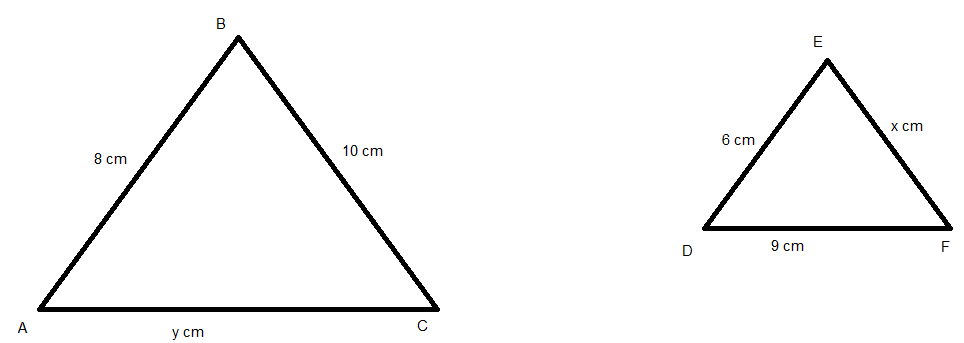CBSE
Mathematics
Criteria for similarity of triangles
A girl of height 90 cm is walking away from the base of a lamp-post at a speed at 1.2 m/sec. If the lamp is 3.6 m above the ground, find the length of her shadow after 4 seconds.
CBSE
Mathematics
Criteria for similarity of triangles
ABCD is a rhombus and P, Q, R, S are the mid-points of the sides AB, BC, CD and DA respectively. Show that the quadrilateral PQRS is a rectangle.
CBSE
Mathematics
Criteria for similarity of triangles
State $AAA$ - similarity criteria ?

CBSE
Mathematics
Criteria for similarity of triangles
ABC and DBC are two triangles on the same base BC. If AD intersects BC at O, show that
$\dfrac{{ar\left( {ABC} \right)}}{{ar\left( {DBC} \right)}} = \dfrac{{AO}}{{DO}}$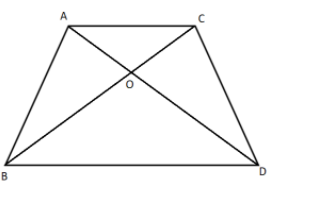CBSE
Maths
Criteria for similarity of triangles
A man of height 1.8m is standing near a pyramid. If the shadow of the man is of length 2.7m and the shadow of the pyramid is 210m long at that instant, find the height of the pyramid.
CBSE
Mathematics
Criteria for similarity of triangles
D, E, F are respectively the mid points of the sides BC, CA and AB of a $\Delta ABC$. Show that
(i) BDEF is a parallelogram
(ii) $ar\left( \Delta DEF \right)=\dfrac{1}{4}ar\left( \Delta ABC \right)$
(iii) $ar\left( BDEF \right)=\dfrac{1}{2}ar\left( \Delta ABC \right)$

CBSE
Mathematics
Criteria for similarity of triangles
Diagonals AC and BD of a trapezium ABCD with $AB\parallel DC$ intersect each other at the point O . Using similarity criterion for two triangles, show that $\dfrac{OA}{OC}=\dfrac{OB}{OD}$.

CBSE
Mathematics
Criteria for similarity of triangles
State which pairs of triangles in figure are similar? Write the similarity criterion used by you for answering the question and also write the pairs of similar triangles in the symbolic form: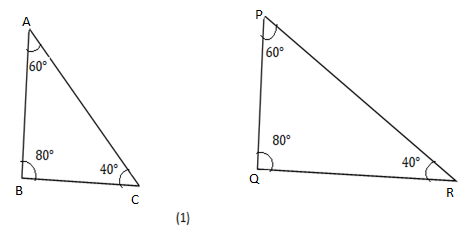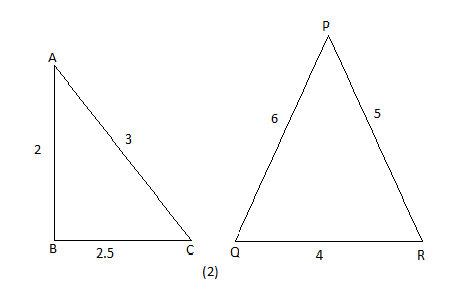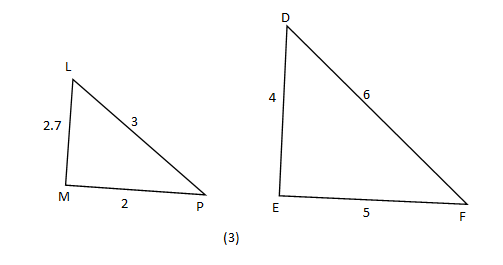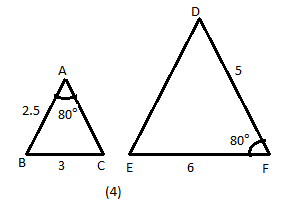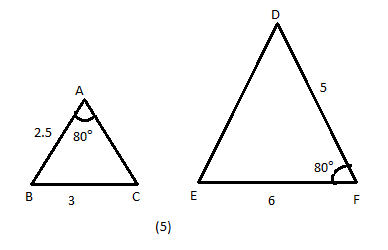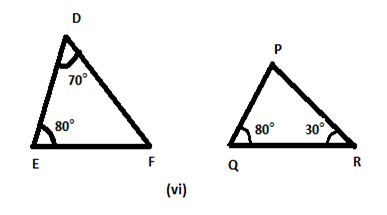CBSE
Mathematics
Criteria for similarity of triangles
In the given figure $E$ is a point on side $CB$ produced of an isosceles triangle $ABC$ with $AB = AC$. If $AD \bot BC$ and $EF \bot AC$ , prove that $\Delta ABD \sim \Delta ECF$.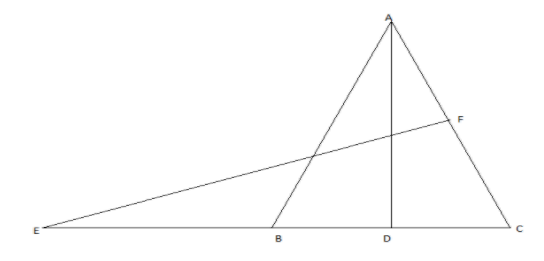CBSE
Mathematics
Criteria for similarity of triangles
If one angle of a triangle is equal to one angle of the other triangle and the sides including these angles are proportional, then the two triangles are similar.

CBSE
Mathematics
Criteria for similarity of triangles
If the triangle ABC is similar to the triangle PQR. Then find the value of PQ.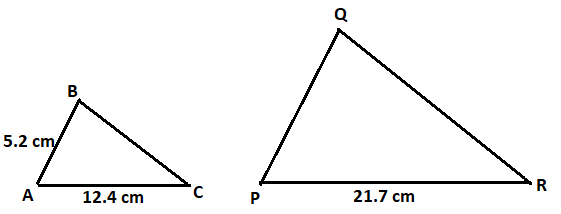Prev
1
2